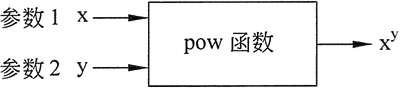## switch语句

### 语法

C++ 中 switch 语句的语法：

cpp
``````
switch(expression){
case constant-expression :
statement(s);
break; // 可选的
case constant-expression :
statement(s);
break; // 可选的

// 您可以有任意数量的 case 语句
default : // 可选的
statement(s);
}
``````

switch语句必须遵循下面的规则：

1. switch 语句中的expression必须是一个整型或枚举类型，或者是一个class类型，其中class 有一个单一的转换函数将其转换为整型或枚举类型。
2. 在一个 switch 中可以有任意数量的 case 语句。每个case后跟一个要比较的值和一个冒号。
3. case 的 constant-expression必须与switch中的变量具有相同的数据类型，且必须是一个常量或字面量。
4. 当被测试的变量等于 case 中的常量时，case 后跟的语句将被执行，直到遇到 break 语句为止。
5. 当遇到 break 语句时，switch 终止，控制流将跳转到 switch 语句后的下一行。
6. 不是每一个 case 都需要包含 break。如果 case 语句不包含 break，控制流将会继续 后续的 case，直到遇到 break 为止。
7. 一个 switch 语句可以有一个可选的 default case，出现在 switch 的结尾。default case 可用于在上面所有 case 都不为真时执行一个任务。default case 中的 break 语句不是必需的。

### 流程图### 嵌套 switch 语句

C++ 中的 switch 语句允许至少 256 个嵌套层次。

cpp
``````
switch(ch1) {
case 'A':
cout << "这个 A 是外部 switch 的一部分";
switch(ch2) {
case 'A':
cout << "这个 A 是内部 switch 的一部分";
break;
case 'B': // 内部 B case 代码
}
break;
case 'B': // 外部 B case 代码
}
``````

## pow()函数

pow函数的作用是求幂。

math.h/cmath

pow(x , y)

### 返回类型

double型。

1. 程序必须包含 cmath 头文件；
2. 在传递给函数的两个参数中，至少第一个参数应该是 double 类型的，当然也可以两个都是；
3. 因为 pow 函数返回一个 double 值，所以被赋值的任何变量也应该是 double 类型的；## sqrt()函数

math.h/cmath

### 功能

double sqrt(double x)## abs()函数

int abs(int x) 返回 x 的绝对值。

int abs(int x)

math.h/cmath

## ceil()函数

### 原型

double ceil(double x)

math.h/cmath

### 原型

double round(double x)

round()用于舍入给定的数字，该数字可以是float或double。它将舍入函数中提供的参数返回最接近的整数值，中间情况舍入为零。

math.h/cmath

## 实例

### 四则运算计算器

2 + 3

5.00

5 / 2

2.50

cpp
``````
#include <iostream>
#include <cstdio>
using namespace std;
int main()
{
double a = 0,b = 0,d = 0;
char c = 0;
cin>>a>>c>>b;
switch(c)
{
case '+':d = a + b;break;
case '-':d = a - b;break;
case '*':d = a * b;break;
case '/':d = a / b;break;
}
printf("%.2lf",d);
return 0;
``````

### 球的体积

10

4186.667

cpp
``````
#include <iostream>
#include <cstdio>
#include <cmath>
using namespace std;
int main()
{
double v = 0,pi = 3.14,r = 0;
cin>>r;
v = 4.0/3*pi*pow(r,3);
printf("%.3lf",v);
return 0;
}
``````

### 恩格尔系数

N = 人均食物支出金额 / 人均总支出金额 * 100%

10 20

cpp
``````
#include <iostream>
#include <cstdio>
using namespace std;
int main()
{
double a = 0,b = 0,n = 0;
cin>>a>>b;
n = a / b * 100;
if (n >= 60)
cout<<"贫穷";
else if (n >= 50 && n < 60)
cout<<"温饱";
else if (n >= 40 && n < 50)
cout<<"小康";
else if (n >= 30 && n < 40)
cout<<"相对富裕";
else if (n >= 20 && n < 30)
cout<<"富裕";
else
cout<<"极其富裕";
return 0;
}
``````

### 计算a、b、c的n次方和

11 22 33 4

1434818

cpp
``````
#include <iostream>
#include <cstdio>
#include <cmath>
using namespace std;
int main()
{
int a = 0,b = 0,c = 0,n = 0,d = 0;
cin>>a>>b>>c>>n;
a = pow(a,n);
b = pow(b,n);
c = pow(c,n);
cout<<a+b+c;
return 0;
}
``````

### 小明买书

37

6元:4 13元:1 15元:0 20元:0

cpp
``````
#include <iostream>
#include <cstdio>
using namespace std;
int main()
{
int x = 0,a = 0,b = 0,c = 0,d = 0;
cin>>x;
a = x / 6;
switch (x % 6)
{
case 1:a -= 2,b += 1;break;
case 2:a -= 3,d += 1;break;
case 3:a -= 2,c += 1;break;
case 4:a -= 4,b += 1,c += 1;break;
case 5:a -= 5,c += 1,d += 1;
}
cout<<a<<" "<<b<<" "<<c<<" "<<d;
return 0;
}
``````